# “EA 交易”运行期间平衡曲线斜率的控制

9 十二月 2013, 13:03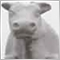0
1 810

### 操作原理• 步进 - 交易量以离散步进从最大到最小以及从最小到最大进行变换；
• 线性 - 交易量根据调节区间内平衡曲线的坡度线性变化；
• 带延迟的步进 - 从最大转换到最小交易量并返回，于不同坡度值处执行。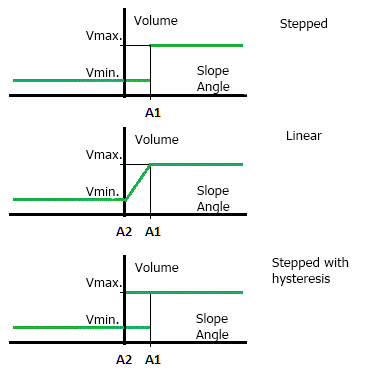• 平衡线斜率的管理效力，直接取决于正常操作模式下有效交易量同交易量拒绝模式下交易量的比率。该率值越大，管理效力越高。正因如此，初始有效交易量应明显大于最低可能交易量。
• “EA 交易”平衡的涨落变更的平均周期，应远大于该控制系统的反应时间。否则，该系统就不能完成平衡曲线斜率的调节。反应时间的平均周期率值越大，系统效力就越大。此要求关系到每一个自动调节系统。

### 利用面向对象编程于 MQL5 中的实施

```//---------------------------------------------------------------------
//  工作交易品种的操作:
//---------------------------------------------------------------------
{
private:

private:
double  max_total_volume;                      // 最大交易量增量
double  symbol_point;                          // 点数大小
double  symbol_tick_size;                      // 最小价格增量
int     symbol_digits;                        // 小数点之后位数

protected:

public:
void    RefreshSymbolInfo( );                  // 刷新工作交易品种的市场情报
void    SetTradeSymbol( string _symbol );      // 设置/改变 工作交易品种
double  GetMaxTotalLots( );                    // 获得最大累积量
double  GetPoints( double _delta );            // 得到价格变化点数

public:
double  NormalizeLots( double _requied_lot );  // 得到归一化交易量
double  NormalizePrice( double _org_price );   // 得到归一化的价格，同时考虑报价的逐步变化

public:
};
```

```//---------------------------------------------------------------------
//  刷新工作交易品种的市场信息:
//---------------------------------------------------------------------
void
{
//  如果工作交易品种未设置, 不做任何事:
if( GetTradeSymbol( ) == NULL )
{
return;
}

//  计算归一化交易量的必要参数:

max_total_volume = SymbolInfoDouble( GetTradeSymbol( ), SYMBOL_VOLUME_LIMIT );

symbol_point = SymbolInfoDouble( GetTradeSymbol( ), SYMBOL_POINT );
symbol_digits = ( int )SymbolInfoInteger( GetTradeSymbol( ), SYMBOL_DIGITS );
}
```

`void    SetTradeSymbol( string _symbol );      // 设置/改变 工作交易品种`

```//---------------------------------------------------------------------
//  获得标准化的交易量:
//---------------------------------------------------------------------
//  - 输入必要的交易量;
//  - 输出标准化交易量;
//---------------------------------------------------------------------
double
{
double   lots, koeff;
int      nmbr;

//  如果工作交易品种未设置, 不做任何事:
if( GetTradeSymbol( ) == NULL )
{
return( 0.0 );
}

{
nmbr = ( int )MathLog10( koeff );
}
else
{
nmbr = 2;
}
lots = MathFloor( _requied_lots * koeff ) / koeff;

//  交易量下限:
{
}

//  交易量上限:
{
}

lots = NormalizeDouble( lots, nmbr );
return( lots );
}
```

```//---------------------------------------------------------------------
//  考虑价格改变步骤的正常价格:
//---------------------------------------------------------------------
double
{
//  每步报价的最小点数:
double  min_price_step = NormalizeDouble( symbol_tick_size / symbol_point, 0 );

double  norm_price = NormalizeDouble( NormalizeDouble(( NormalizeDouble( _org_price / symbol_point, 0 )) / min_price_step, 0 ) * min_price_step * symbol_point, symbol_digits );
return( norm_price );
}
```

### TBalanceHistory 类

```//---------------------------------------------------------------------
//  结余历史的操作:
//---------------------------------------------------------------------
class TBalanceHistory
{
private:
long      current_magic;            // 当存取历史合约时的 "幻数"  ( 0 - 任意数字 )
long      current_type;             // 合约类型 ( -1 - 全部 )
int       current_limit_history;   // 历史深度限制 ( 0 - 所有历史 )
datetime   monitoring_begin_date;   // 监视合约历史的开始时间

protected:

protected:
//  "原始" 数组:
double    org_datetime_array[ ];                                                                                                                                                      // 交易日期时间
double    org_result_array[ ];                                                                                                                                                                // 交易结果

//  以时间归组的数据数组:
double    group_datetime_array[ ];                                                                                                                                            // 交易日期时间
double    group_result_array[ ];                                                                                                                                                      // 交易结果

double    last_result_array[ ];     // 保存最后交易结果的数组 ( Y 轴上的点数 )
double    last_datetime_array[ ];   // 存最后交易时间的数组 ( X 轴上的点数 )

private:
void      SortMasterSlaveArray( double& _m[ ], double& _s[ ] );  // 同步两个升序数组

public:
void      SetTradeSymbol( string _symbol );                      // 设置/改变 工作交易品种
void      RefreshSymbolInfo( );                                 // 刷新工作交易品种的市场情报
void      SetMonitoringBeginDate( datetime _dt );                // 设置监视开始时间
datetime  GetMonitoringBeginDate( );                            // 获取监视开始时间
void      SetFiltrParams( long _magic, long _type = -1, int _limit = 0 );// 设置合约过滤参数

public:
// 得到最后交易结果:

public:
void      TBalanceHistory( );       // 构造器
void      ~TBalanceHistory( );      // 析构器
};
```

• _magic           - 应于历史中读取的交易“幻数”。如果指定零值，则带有任何“幻数”的交易都会被读取。
• _type             - 应被读取的交易类型。可拥有下述值 - DEAL_TYPE_BUY （仅限读取长线交易）， DEAL_TYPE_SELL （仅限读取短线交易）以及 -1 （读取长线短线两种交易）。
• _limit             - 限制被分析交易历史的深度。如其等于零，则所有可用历史均被分析。

```//---------------------------------------------------------------------
//  读取数组最近（按时间）交易的结果:
//---------------------------------------------------------------------
//  - 返回实际读取交易数量但不超过指定数量;
//---------------------------------------------------------------------
int
{
int       index, limit, count;
long      deal_type, deal_magic, deal_entry;
datetime   deal_close_time, current_time;
ulong     deal_ticket;                        // 合约的单号
string    symbol, deal_symbol;

//  交易数量不得少于 2:
{
return( 0 );
}

//  如果工作交易品种未指定, 不做任何事:
if( symbol == NULL )
{
return( 0 );
}

//  请求从指定时间至当前时刻的历史合约与订单:
if( HistorySelect( monitoring_begin_date, TimeCurrent( )) != true )
{
return( 0 );
}

//  计算交易数量:
count = HistoryDealsTotal( );

//  如果历史交易数量少于必要数量, 则退出:
{
return( 0 );
}

//  如果历史交易数量多于必要数量, 则限制它们:
if( current_limit_history > 0 && count > current_limit_history )
{
limit = count - current_limit_history;
}
else
{
limit = 0;
}

//  如果需要, 调整 "原始" 数组的维度至指定的交易数量:
if(( ArraySize( org_datetime_array )) != ( count - limit ))
{
ArrayResize( org_datetime_array, count - limit );
ArrayResize( org_result_array, count - limit );
}

//  以历史交易填充 "原始" 数组:
for( index = count - 1; index >= limit; index-- )
{
deal_ticket = HistoryDealGetTicket( index );

//  如果合约未平仓, 不要继续:
deal_entry = HistoryDealGetInteger( deal_ticket, DEAL_ENTRY );
if( deal_entry != DEAL_ENTRY_OUT )
{
continue;
}

//  检查合约的 "幻数"，如果必要:
deal_magic = HistoryDealGetInteger( deal_ticket, DEAL_MAGIC );
if( current_magic != 0 && deal_magic != current_magic )
{
continue;
}

//  检查合约交易品种:
deal_symbol = HistoryDealGetString( deal_ticket, DEAL_SYMBOL );
if( symbol != deal_symbol )
{
continue;
}

//  检查合约类型，如果必要:
deal_type = HistoryDealGetInteger( deal_ticket, DEAL_TYPE );
if( current_type != -1 && deal_type != current_type )
{
continue;
}
else if( current_type == -1 && ( deal_type != DEAL_TYPE_BUY && deal_type != DEAL_TYPE_SELL ))
{
continue;
}

//  检查合约平仓时间:
deal_close_time = ( datetime )HistoryDealGetInteger( deal_ticket, DEAL_TIME );
if( deal_close_time < monitoring_begin_date )
{
continue;
}

//  所以, 我们可以读其它交易:
org_datetime_array[ real_trades ] = deal_close_time / 60;
org_result_array[ real_trades ] = HistoryDealGetDouble( deal_ticket, DEAL_PROFIT ) / HistoryDealGetDouble( deal_ticket, DEAL_VOLUME );
}

//  如果此时少于必要交易数, 返回:
{
return( 0 );
}

//  以订单关闭时间对 "原始" 数组排序:
SortMasterSlaveArray( org_datetime_array, org_result_array );

// 如果必要, 调整 group 数组的维度至指定的点数:
if(( ArraySize( group_datetime_array )) != count )
{
ArrayResize( group_datetime_array, count );
ArrayResize( group_result_array, count );
}
ArrayInitialize( group_datetime_array, 0.0 );
ArrayInitialize( group_result_array, 0.0 );

//  以分组数据填充输出数组 ( 平仓时间为标识的分组 ):
for( index = 0; index < count; index++ )
{
//  得到其它交易:
deal_close_time = ( datetime )org_datetime_array[ index ];

//  现在检查是否在输出数组中存在时间相同:
current_time = ( datetime )group_datetime_array[ real_trades ];
if( current_time > 0 && MathAbs( current_time - deal_close_time ) > 0.0 )
{
}
else
{
}
}

//  如果此时少于必要交易数, 退出:
{
return( 0 );
}

if( ArraySize( last_result_array ) != _max_trades )
{
}

//  以逆向索引写积累的数据到输出数组:
for( index = 0; index < _max_trades; index++ )
{
last_result_array[ _max_trades - 1 - index ] = group_result_array[ index ];
last_datetime_array[ _max_trades - 1 - index ] = group_datetime_array[ index ];
}

//  在输出数组中，以积累的总和取代单个交易的结果:
for( index = 1; index < _max_trades; index++ )
{
last_result_array[ index ] += last_result_array[ index - 1 ];
}

}
```

```//  请求从指定时间至当前时刻的历史合约与订单:
if( HistorySelect( monitoring_begin_date, TimeCurrent( )) != true )
{
return( 0 );
}

//  计算交易数量:
count = HistoryDealsTotal( );

//  如果历史交易数量少于必要数量, 则退出:
{
return( 0 );
}
```

```//  以历史交易填充 "原始" 数组:
for( index = count - 1; index >= limit; index-- )
{
deal_ticket = HistoryDealGetTicket( index );

//  如果交易未关闭, 不要继续:
deal_entry = HistoryDealGetInteger( deal_ticket, DEAL_ENTRY );
if( deal_entry != DEAL_ENTRY_OUT )
{
continue;
}

//  检查合约的 "幻数"，如果必要:
deal_magic = HistoryDealGetInteger( deal_ticket, DEAL_MAGIC );
if( _magic != 0 && deal_magic != _magic )
{
continue;
}

//  检查合约交易品种:
deal_symbol = HistoryDealGetString( deal_ticket, DEAL_SYMBOL );
if( symbol != deal_symbol )
{
continue;
}

//  检查合约类型，如果必要:
deal_type = HistoryDealGetInteger( deal_ticket, DEAL_TYPE );
if( _type != -1 && deal_type != _type )
{
continue;
}
else if( _type == -1 && ( deal_type != DEAL_TYPE_BUY && deal_type != DEAL_TYPE_SELL ))
{
continue;
}

//  检查合约平仓时间:
deal_close_time = ( datetime )HistoryDealGetInteger( deal_ticket, DEAL_TIME );
if( deal_close_time < monitoring_begin_date )
{
continue;
}

//  所以, 我们可以读其它交易:
org_datetime_array[ real_trades ] = deal_close_time / 60;
org_result_array[ real_trades ] = HistoryDealGetDouble( deal_ticket, DEAL_PROFIT ) / HistoryDealGetDouble( deal_ticket, DEAL_VOLUME );
}

//  如果此时少于必要交易数, 退出:
{
return( 0 );
}

```

```//  检查合约平仓时间:
deal_close_time = ( datetime )HistoryDealGetInteger( deal_ticket, DEAL_TIME );
if( deal_close_time < monitoring_begin_date )
{
continue;
}

```

TBalanceHistory::SortMasterSlaveArray( double& _master[ ], double& _slave[ ] )。第一个参数是 _master - 按升序排列的一个数组。第二个参数为 _slave - 数组的元素应与第一个数组元素同时移动。排序则通过 "bubble" 方法来实现。

```//  以分组数据填充输出数组 ( 平仓时间为标识的分组 ):
for( index = 0; index < count; index++ )
{
//  得到其它交易:
deal_close_time = ( datetime )org_datetime_array[ index ];

//  现在检查是否在输出数组中存在时间相同:
current_time = ( datetime )group_datetime_array[ real_trades ];
if( current_time > 0 && MathAbs( current_time - deal_close_time ) > 0.0 )
{
}
else
{
}
}

``````//  以逆向索引写积累的数据到输出数组:
for( index = 0; index < _max_trades; index++ )
{
last_result_array[ _max_trades - 1 - index ] = group_result_array[ index ];
last_datetime_array[ _max_trades - 1 - index ] = group_datetime_array[ index ];
}

//  在输出数组中，以积累的总和取代单个交易的结果:
for( index = 1; index < _max_trades; index++ )
{
last_result_array[ index ] += last_result_array[ index - 1 ];
}

```

### TBalanceSlope 类

```//---------------------------------------------------------------------
//  结余曲线操作:
//---------------------------------------------------------------------
class TBalanceSlope : public TBalanceHistory
{
private:
double    current_slope;               // 结余曲线的斜率的当前角度
int       slope_count_points;          // 计算斜率角度的点数 ( 交易 )

private:
double    LR_koeff_A, LR_koeff_B;      // 直线回归方程的比率
double    LR_points_array[ ];          // 直线回归方程点的数组

private:
void      CalcLR( double& X[ ], double& Y[ ] );  // 计算直线回归方程

public:
void      SetSlopePoints( int _number );        // 设置计算斜率角度的点数
double    CalcSlope( );                         // 计算斜率角度

public:
void      TBalanceSlope( );                     // 构造器
void      ~TBalanceSlope( );                    // 析构器
};
```

```//---------------------------------------------------------------------
//  计算直线回归方程式:
//---------------------------------------------------------------------
//  输入参数:
//    X[ ] - X轴数列值;
//    Y[ ] - Y轴数量值;
//---------------------------------------------------------------------
void
TBalanceSlope::CalcLR( double& X[ ], double& Y[ ] )
{
double    mo_X = 0, mo_Y = 0, var_0 = 0, var_1 = 0;
int       i;
int       size = ArraySize( X );
double    nmb = ( double )size;

//  如果点数小于 2, 则曲线不可计算:
if( size < 2 )
{
return;
}

for( i = 0; i < size; i++ )
{
mo_X += X[ i ];
mo_Y += Y[ i ];
}
mo_X /= nmb;
mo_Y /= nmb;

for( i = 0; i < size; i++ )
{
var_0 += ( X[ i ] - mo_X ) * ( Y[ i ] - mo_Y );
var_1 += ( X[ i ] - mo_X ) * ( X[ i ] - mo_X );
}

//  A 值的系数:
if( var_1 != 0.0 )
{
LR_koeff_A = var_0 / var_1;
}
else
{
LR_koeff_A = 0.0;
}

//  B 值的系数:
LR_koeff_B = mo_Y - LR_koeff_A * mo_X;

//  填充附在回归线上的点数组:
ArrayResize( LR_points_array, size );
for( i = 0; i < size; i++ )
{
LR_points_array[ i ] = LR_koeff_A * X[ i ] + LR_koeff_B;
}
}
```

```//---------------------------------------------------------------------
//  计算斜角:
//---------------------------------------------------------------------
double
TBalanceSlope::CalcSlope( )
{
//  从历史交易中取得交易结果:
int      nmb = GetTradeResultsArray( slope_count_points );
if( nmb < slope_count_points )
{
return( 0.0 );
}

//  以最后交易结果计算回归线:
CalcLR( last_datetime_array, last_result_array );
current_slope = LR_koeff_A;

return( current_slope );
}
```

### TBalanceSlopeControl 类

```//---------------------------------------------------------------------
//  管理结余曲线的倾斜率:
//---------------------------------------------------------------------
enum LotsState
{
LOTS_NORMAL = 1,            // 正常交易量的交易模式
LOTS_REJECTED = -1,         // 低交易量的交易模式
LOTS_INTERMEDIATE = 0,      // 中间交易量的交易模式
};
//---------------------------------------------------------------------
class TBalanceSlopeControl : public TBalanceSlope
{
private:
double    min_slope;          // 对应交易量拒绝模式的倾角
double    max_slope;          // 对应交易量正常模式的倾角
double    centr_slope;        // 对应交易量无迟滞切换模式的倾角

private:
ControlType  control_type;    // 调节功能的类型

private:
double    rejected_lots;      // 交易量拒绝模式
double    normal_lots;        // 交易量正常模式
double    intermed_lots;      // 交易量中间模式

private:
LotsState current_lots_state; // 当前交易量模式

public:
void      SetControlType( ControlType _control );  // 设置调节特征类型
void      SetControlParams( double _min_slope, double _max_slope, double _centr_slope );

public:
double    CalcTradeLots( double _min_lots, double _max_lots );  // 获取交易量

protected:
double    CalcIntermediateLots( double _min_lots, double _max_lots, double _slope );

public:
void      TBalanceSlopeControl( );   // 构造器
void      ~TBalanceSlopeControl( );  // 析构器
};
```

```  void      SetControlType( ControlType _control );  // 设置调节特征类型

```

Input parameter_control - 此为调节特性的类型。它可以拥有下述值：

• STEP_WITH_HYSTERESISH      - 带有延迟调节的步进特性；
• STEP_WITHOUT_HYSTERESIS  - 不带有延迟调节的步进特性；
• LINEAR                                   - 线性调节特性；
• NON_LINEAR                           - 非线性调节特性（未于本版本中实现）；

```  void      SetControlParams( double _min_slope, double _max_slope, double _centr_slope );

```

• _min_slope - 与带有最小交易量的交易对应的平衡曲线坡度；
• _max_slope - 与带有最大交易量的交易对应的平衡曲线坡度；
• _centr_slope - 与不带延迟步进调节特性对应的平衡曲线坡度；

```//---------------------------------------------------------------------
//  获得交易量:
//---------------------------------------------------------------------
double
TBalanceSlopeControl::CalcTradeLots( double _min_lots, double _max_lots )
{
//  尝试计算结余曲线的斜率:
double    current_slope = CalcSlope( );

//  如果指定交易额度不能积累, 以最小的交易量:
if( GetRealTrades( ) < GetSlopePoints( ))
{
current_lots_state = LOTS_REJECTED;
return( rejected_lots );
}

// 如果调节功能无迟滞:
if( control_type == STEP_WITHOUT_HYSTERESIS )
{
if( current_slope < centr_slope )
{
current_lots_state = LOTS_REJECTED;
return( rejected_lots );
}
else
{
current_lots_state = LOTS_NORMAL;
return( normal_lots );
}
}

//  如果结余曲线的线形回归斜率小于许可:
if( current_slope < min_slope )
{
current_lots_state = LOTS_REJECTED;
return( rejected_lots );
}

//  如果结余曲线的线形回归斜率大于指定:
if( current_slope > max_slope )
{
current_lots_state = LOTS_NORMAL;
return( normal_lots );
}

//  如果结余曲线的线形回归斜率在边界之内 (中间状态):
current_lots_state = LOTS_INTERMEDIATE;

//  计算中间交易量的值:
intermed_lots = CalcIntermediateLots( _min_lots, _max_lots, current_slope );

return( intermed_lots );
}
```

• 直至累积到指定的最小交易量，再以最小交易量交易。这样合情合理，因为在您刚刚设置好的时候，“EA 交易”所处的周期（是否可盈利）还都是未知。
• 如果是不带延迟步进的调节函数，则通过 TBalanceSlopeControl::SetControlParams 方法设置交易模式之间的切换角度，只能采用 _centr_slope 参数。_min_slope_max_slope 参数被忽略。为此，要通过 MetaTrader 5 策略测试程序中的这个参数来执行正确优化。

```//---------------------------------------------------------------------
//  计算中间交易量:
//---------------------------------------------------------------------
double
TBalanceSlopeControl::CalcIntermediateLots( double _min_lots, double _max_lots, double _slope )
{
double    lots;

// 如果调节功能迟滞:
if( control_type == STEP_WITH_HYSTERESISH )
{
if( current_lots_state == LOTS_REJECTED && _slope > min_slope && _slope < max_slope )
{
lots = _min_lots;
}
else if( current_lots_state == LOTS_NORMAL && _slope > min_slope && _slope < max_slope )
{
lots = _max_lots;
}
}
// 如果调节功能是线性:
else if( control_type == LINEAR )
{
double  a = ( _max_lots - _min_lots ) / ( max_slope - min_slope );
double  b = normal_lots - a * .max_slope;
lots = a * _slope + b;
}
// 如果调节功能是非线性 (尚未实现):
else if( control_type == NON_LINEAR )
{
lots = _min_lots;
}
// 如果调节功能未知:
else
{
lots = _min_lots;
}

return( lots );
}
```

### 将此系统嵌入“EA 交易”示例

`#include  <BalanceSlopeControl.mqh>`

```//---------------------------------------------------------------------
//  控制结余曲线倾斜率的系统的参数;
//---------------------------------------------------------------------
enum SetLogic
{
No = 0,
Yes = 1,
};
//---------------------------------------------------------------------
input SetLogic     UseAutoBalanceControl = No;
//---------------------------------------------------------------------
input ControlType  BalanceControlType = STEP_WITHOUT_HYSTERESIS;
//---------------------------------------------------------------------
//  最后交易额度用于计算结余曲线的 LR:
//---------------------------------------------------------------------
//  LR 斜率，降低交易量至最小:
input double       LRKoeffForRejectLots = -0.030;
//---------------------------------------------------------------------
//  LR 斜率，恢复正常交易模式:
input double       LRKoeffForRestoreLots = 0.050;
//---------------------------------------------------------------------
//  LR 斜率，工作在中间模式:
input double       LRKoeffForIntermedLots = -0.020;
//---------------------------------------------------------------------
//  降低初始交易量至指定值，当 LR 倾斜向下
input double       RejectedLots = 0.10;
//---------------------------------------------------------------------
//  正常工作交易量，在资金管理为固定交易量模式:
input double       NormalLots = 1.0;
```

`TBalanceSlopeControl  BalanceControl;`

```//  调整我们控制结余曲线斜率的系统:
BalanceControl.SetControlType( BalanceControlType );
BalanceControl.SetControlParams( LRKoeffForRejectLots, LRKoeffForRestoreLots, LRKoeffForIntermedLots );
BalanceControl.SetFiltrParams( 0, -1, 0 );
BalanceControl.SetMonitoringBeginDate( 0 );
```

```//  刷新市场情报:
BalanceControl.RefreshSymbolInfo( );
```

```  if( UseAutoBalanceControl == Yes )
{
current_lots = BalanceControl.CalcTradeLots( RejectedLots, NormalLots );
}
else
{
current_lots = NormalLots;
}
```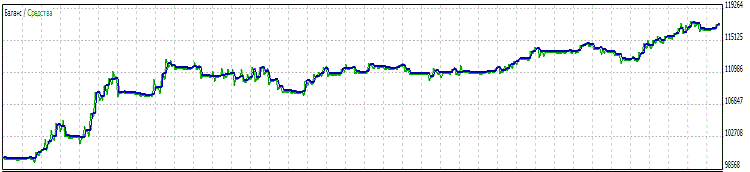参数
UseAutoBalanceControl = No  UseAutoBalanceControl = Yes

平衡绝对减值： 1 310.50 131.05
资产净值绝对减值：  1 390.50 514.85
平衡最大减值：  5 569.50 (5.04%) 3 762.15 (3.35%)
资产净值最大减值： 6 899.50 (6.19%)
4 609.60 (4.08%)

### 总结

• 在“EA 交易”进入某个不利运行周期时，使用虚拟交易。如此一来，不会对正常的有效交易量造成任何影响。它还能降低减值。
• 采用更多复杂的算法来确定“EA 交易”运行的当前状态（是否盈利）。比如说，我们可以尝试针对此分析应用一个神经网络。当然，这种情况也需要更进一步的探索。

bscs-testexpert.mq5 (15.77 KB)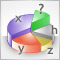通过指定的幻数计算总持仓量的最佳方法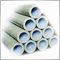一个使用命名管道在 MetaTrader 5 客户端之间进行通信的无 DLL 解决方案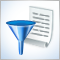查找错误和记录

MetaEditor 5 具备调试功能。但是在编写 MQL5 程序时，您通常都希望不要显示个别的值，而是测试与在线工作期间出现的所有信息。如果日志文件内容庞大，所需信息快速便捷检索自动化的重要性就显而易见了。本文中，我们会研究 MQL5 程序中查找错误的方式以及记录方法。我们也会简单地记录到文件中，并了解一款方便日志查看的简单程序 - LogMon。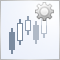New Bar (新柱)事件处理程序

MQL5 编程语言处理问题的能力已达到一个全新的水平。即便是那些已有此类解决方案的任务，也因为面向对象编程而进阶到一个更高的水平。本文中，我们会举一个检查图表中新柱的特别简单的例子，而且，它已经转化成为一种相当强大且用途多样的工具。什么工具？到文中找答案吧。